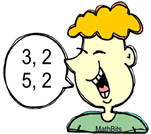Factoring Trinomials Practice Terms of Use    Contact Person: Donna RobertsDirections: Answer these questions pertaining to factoring. Only completely factored answers are deemed as correct. The cartoon people may, or may not, be helpful!! Check each answer when finished.

1.
Factor completely:   x2 + 9x + 14

Choose:
 (x + 7)(x + 2) (x - 2)(x - 7) (x + 14)(x + 1) (x - 14)(x - 1)2.
Factor completely:   2x3 - 14x2 + 24x

Choose:
 x(2x - 8)(x - 3) (2x2 - 8x)(x - 3) 2x(x + 4)(x + 3) 2x(x - 4)(x - 3)3.
Factor completely:   ax2 - 3ax - 54a

Choose:
 a(x2 - 3x - 54) x(a2 - 3a - 54) a(x + 6)(x - 9) x(a + 6)(a - 9)4.
Factor completely:   6x2 + 11x - 10
Choose:
 2(x + 1)(3x - 5) (3x + 2)(2x - 5) (6x - 2)(x + 5) (3x - 2)(2x + 5)5.
Factor completely:    y4 + y2 - 12

Choose:
 y2(y - 3)(y + 4) (y2 - 3)(y2 + 4) y2(y + 3)(y - 4) (y2 + 3)(y2 - 4)6.
Factor completely:    -8x3 - 18x2 - 4x

Choose:
 2x(x + 2)(-4x + 1) -2x(-x - 2)(4x + 1) -2x(x + 2)(4x + 1) 2x(-x + 2)(-4x + 1)7.
Factor completely:    5a2 - 11a + 6

Choose:
 (5a - 3)(a - 2) (5a - 2)(a - 3) (5a - 1)(a - 6) (5a - 6)(a - 1)8.
Factor completely:   m2 - 12m + 36

Choose:
 (m + 6)(m - 6) (m - 6)2 (m + 6)2 (m - 12)(m + 3)9.
Factor completely:   x2 - 6x - 19

Choose:
 (x - 19)(x + 1) (x - 3)(x + 2) (x + 19)(x - 1) prime (no integer factors)10.
Factor completely:   -m2 + 18m - 45

Choose:
 -(m - 3)(m + 15) -(m + 3)(m + 15) -(m - 3)(m - 15) (m - 3)(m - 15)